Courses

# Introduction to Physics NEET Notes | EduRev

## JEE : Introduction to Physics NEET Notes | EduRev

The document Introduction to Physics NEET Notes | EduRev is a part of the JEE Course HC Verma and Irodov Solutions.
All you need of JEE at this link: JEE

Page No 8:

Ques 1: The metre is defined as the distance travelled by light in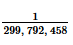second. Why didn't people choose some easier number such as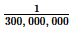second? Why not 1 second?
Ans: The speed of light in vacuum is 299,792,458 m/s.
Then time taken by light to cover a distance of 1 metre in vacuum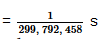Hence, the metre is defined as the distance travelled by light in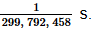As 300,000,000 m/s is an approximate speed of light in vacuum, it cannot be used to define the metre.
The distance travelled by light in one second is 299,792,458 m. This is a large quantity and cannot be used as a base unit. So, the metre is not defined in terms of second.

Ques 2: What are the dimensions of:
(a) volume of a cube of edge a,
(b) volume of a sphere of radius a,
(c) the ratio of the volume of a cube of edge a to the volume of a sphere of radius a?

Ans: (a) Volume of a cube of edge a, V=a x a x a
i.e., [V] = L x L x L = L3
(b) Volume of a sphere of radius a,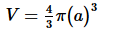i.e., [V] = L x L x L = L3

(c) The ratio of the volume of the cube to the volume of the sphere is a dimensionless quantity.

#### Page No 9:

Ques 1: Suppose you are told that the linear size of everything in the universe has been doubled overnight. Can you test this statement by measuring sizes with a metre stick? Can you test it by using the fact that the speed of light is a universal constant and has not changed? What will happen if all the clocks in the universe also start running at half the speed?
Ans: The validity of this statement cannot be tested by measuring sizes with a metre stick, because the size of the metre stick has also got doubled overnight.
Yes, it can be verified by using the fact that speed of light is a universal constant and has not changed.
If the linear size of everything in the universe is doubled and all the clocks in the universe starts running at half the speed, then we cannot test the validity of this statement by any method.

Ques 2: If all the terms in an equation have same units, is it necessary that they have same dimensions? If all the terms in an equation have same dimensions, is it necessary that they have same units?
Ans: Yes, if all the terms in an equation have the same units, it is necessary that they have the same dimension.
No, if all the terms in an equation have the same dimensions, it is not necessary that they have the same unit. It is because two quantities with different units can have the same dimension, but two quantities with different dimensions cannot have the same unit. For example angular frequency and frequency, both have the dimensions [T-1] but units of angular frequency is rad/s and frequency is Hertz. Another example is energy per unit volume and pressure.Both have the dimensions of [ML-1T-2] but units of pressure is N/m2 and that of energy per unit volume is J/m3.

Ques 3: If two quantities have same dimensions, do they represent same physical content?
Ans: No, even if two quantities have the same dimensions, they may represent different physical contents.
Example: Torque and energy have the same dimension, but they represent different physical contents.

Ques 4: It is desirable that the standards of units be easily available, invariable, indestructible and easily reproducible. If we use foot of a person as a standard unit of length, which of the above features are present and which are not?
Ans: If we use the foot of a person as a standard unit of length,features that  will not be present are  variability, destructibility and  reproducible nature and the feature that will be present is the availability of  the foot of a person to measure any length.

Ques 5: Suggest a way to measure:
(a) the thickness of a sheet of paper,
(b) the distance between the sun and the moon.

Ans: (a) The thickness of a sheet of paper can roughly be determined by measuring the height of a stack of paper.
Example: Let us consider a stack of 100 sheets of paper. We will use a ruler to measure its height. In order to determine the thickness of a sheet of paper, we will divide the height of the stack with the number of sheets (i.e., 100).
(b) The distance between the Sun and the Moon can be measured by using Pythagoras theorem when the Earth makes an angle of 90  with the Sun and the Moon. We already know the distances from the Sun to the Earth and from the Earth to the Moon. However, these distances keep on changing due to the revolution of the Moon around the Earth and the revolution of the Earth around the Sun.

Ques 6: Which of the following sets cannot enter into the list of fundamental quantities in any system of units?
(a) length, mass and velocity,
(b) length, time and velocity,
(c) mass, time and velocity,
(d) length, time and mass.

Ans: (b) length, time and velocity
We define length and time separately as it is not possible to define velocity without using these quantities. This means that one fundamental quantity depends on the other. So, these quantities cannot be listed as fundamental quantities in any system of units.

Ques 7: A physical quantity is measured and the result is expressed as nu where u is the unit used and n is the numerical value. If the result is expressed in various units then
(a)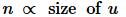(b)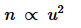(c)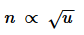(d)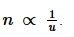Ans:  (d)The larger the unit used to express the physical quantity, the lesser will be the numerical value.
Example: 1 kg of sugar can be expressed as 1000 g or 10000 mg of sugar.
Here, g (gram) is the larger quantity as compared to mg (milligram), but the numerical value used with gram is lesser than the numerical value used with milligram.

Ques 8: Suppose a quantity x can be dimensionally represented in terms of M, L and T, that is, [x] = MaLbT. The quantity mass

(a) can always be dimensionally represented in terms of L, T and x,
(b) can never be dimensionally represented in terms of L, T and x,
(c) may be represented in terms of L, T and x if a = 0,
(d) may be represented in terms of L, T and x if a ≠ 0.

Ans: (d) may be represented in terms of L, T and x if a ≠ 0.

If a = 0, then we cannot represent mass dimensionally in terms of L, T and x, otherwise it can be represented in terms of L, T and x.

Ques 9: A dimensionless quantity
(a) never has a unit,
(b) always has a unit,
(c) may have a unit,
(d) does not exist.

Ans: (c) may have a unit
Dimensionless quantities may have units.

Ques 10: A unitless quantity
(a) never has a non-zero dimension,
(b) always has a non-zero dimension,
(c) may have a non-zero dimension,
(d) does not exist.

Ans: (a) never has a non-zero dimension
A unitless quantity never has a non-zero dimension.

Ques 11: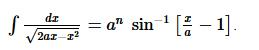The value of n is
(a) 0
(b) −1
(c) 1
(d) none of these.
You may use dimensional analysis to solve the problem.

Ans: (a) 0

[ax] = [x2]

⇒ [a] =[x]  ..(1)

Dimension of LHS = Dimension of RHS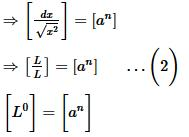n=0

Ques 12: The dimensions ML−1 T−2 may correspond to
(a) work done by a force
(b) linear momentum
(c) pressure
(d) energy per unit volume.

Ans: (c) pressure
(d) energy per unit volume
[Work done] = [ML2T−2]
[Linear momentum] = [MLT−1]
[Pressure] = [ML−1 T−2]
[Energy per unit volume] = [ML−1 T−2]
From the above, we can see that pressure and energy per unit volume have the same dimension, i.e., ML−1 T−2.

Ques 13: Choose the correct statements(s):
(a) A dimensionally correct equation may be correct.
(b) A dimensionally correct equation may be incorrect.
(c) A dimensionally incorrect equation may be correct.
(d) A dimensionally incorrect equation may be incorrect.

Ans: (a) A dimensionally correct equation may be correct.
(b) A dimensionally correct equation may be incorrect.
(d) A dimensionally incorrect equation may be incorrect.
It is not possible that a dimensionally incorrect equation is correct. All the other situations are possible.

Ques 14: Choose the correct statements(s):
(a) All quantities may be represented dimensionally in terms of the base quantities.
(b) A base quantity cannot be represented dimensionally in terms of the rest of the base quantities.
(c) The dimensions of a base quantity in other base quantities is always zero.
(d) The dimension of a derived quantity is never zero in any base quantity.

Ans: The statements which are correct are:
(a) All quantities may be represented dimensionally in terms of the base quantities.
(b) A base quantity cannot be represented dimensionally in terms of the rest of the base quantities.
(c) The dimensions of a base quantity in other base quantities is always zero.
Statement (d) is not correct because A derived quantity can exist which is dimensionless for example fine structure constant which is given by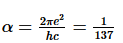where e is the electric charge and c is the speed of light and h is Planks constant.

α is the derived quantity and is dimensionless.

Ques 15: Find the dimensions of
(a) linear momentum,
(b) frequency and
(c) pressure.

Ans: (a) Linear momentum = mv
Here, [m] = [M] and [v] = [LT−1]
∴ Dimension of linear momentum, [mv] = [MLT−1]
(b) Frequency =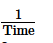∴ Dimension of frequence =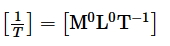(c) Pressure =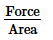Dimension of force = [MLT-2]

Dimension of area = [L2]

∴ Dimension of Pressure =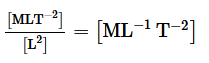Ques 16: Find the dimensions of
(a) angular speed ω,
(b) angular acceleration α,
(c) torque τ and
(d) moment of interia I.
Some of the equations involving these quantities are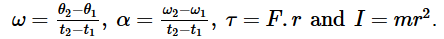The symbols have standard meanings.
Ans: (a) Dimensions of angular speed,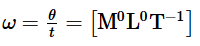(b) Angular acceleration,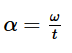Here, ω =[M0L0T-1] and t=[T]

So, dimensions of angular acceleration = [M0L0T−2]

(c) Torque, τ =Frsinθ

Here, F = [MLT−2] and r = [L]
So, dimensions of torque = [ML2T−2]
(d) Moment of inertia = mr2
Here, m = [M] and r2 = [L2]
So, dimensions of moment of inertia = [ML2T0

#### Page No 10:

Ques 1: Find the dimensions of
(a) electric field E.
(b) magnetic field B and
(c) magnetic permeability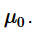The relevant equation are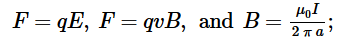where F is force, q is charge, v is speed, I is current, and a is distance.
Ans: (a) Electric field is defined as electric force per unit charge.
i.e.,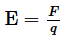Also, [F] = [MLT-2] and [q] = [AT]

So, dimension of elelctric field, [E] = [MLT-3A-1]

(b) Magnetic field,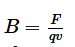Here ,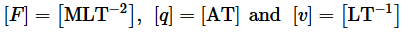So, dimension of magnetic field,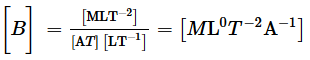(c) Magnetic permeability,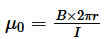Here, [B] = [MT-2A-1] and [r] = [L]

So, dimension of magnetic permeability,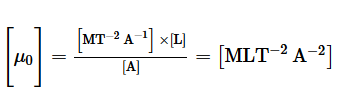Ques 2: Find the dimensions of
(a) electric dipole moment p and
(b) magnetic dipole moment M.
The defining equations are p = q.d and M = IA;
where d is distance, A is area, q is charge and I is current.

Ans: (a) Electric dipole moment, P = q.(2d)
Here, [q] = [AT] and d = [L]
∴ Dimension of electric dipole moment = [LTA]
(b) Magnetic dipole moment, M = IA
Here, A = [L2]
∴ Dimension of magnetic dipole moment = [L2A]

Ques 3: Find the dimensions of Planck's constant h from the equation E = hv where E is the energy and v is the frequency.
Ans: E = hv, where E is the energy and v is the frequency

Here, [E]=[ML2T−2] and [v]=[T−1]

So,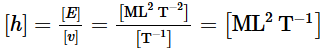Ques 4: Find the dimensions of
(a) the specific heat capacity c,
(b) the coefficient of linear expansion α and
(c) the gas constant R.
Some of the equations involving these quantities are

Q=mc(T2−T1), lt=l0[1+α(T2−T1)] and PV=nRT.
Ans: (a) Specific heat capacity,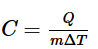[Q]=[ML2T−2] and [T]=[K]

So,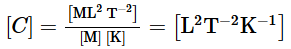(b) Coefficient of linear expansion,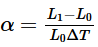So,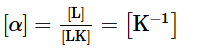(c) Gas constant,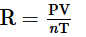Here,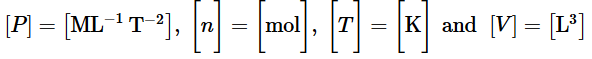So,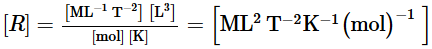Ques 5: Taking force, length and time to be the fundamental quantities, find the dimensions of
(a) density,
(b) pressure,
(c) momentum and
(d) energy.

Ans: Let F be the dimension of force.

(a) Density =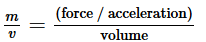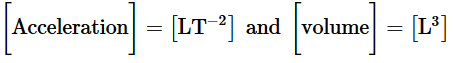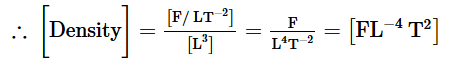(b) Pressure=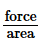[Area]=[L2]

∴ [Pressure]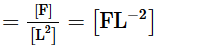(c) Momentum = mv = (force/acceleration) × velocity

Acceleration =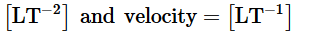∴ [Momentum]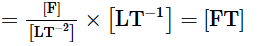(d) Energy =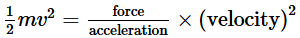∴ [Energy] =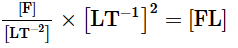Ques 6: Suppose the acceleration due to gravity at a place is 10 m/s2. Find its value if cm/(minute)2
Ans: Acceleration due to gravity, g = 10 m/s2

g = 10 m/s2 = 10 × 100 cm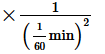g = 1000 × 3600 cm/min2 = 36 × 105 cm/min2

Ques 7: The average speed of a snail is 0⋅020 miles/ hour and that of a leopard is 70 miles/ hour. Convert these speeds in SI units.
Ans: 1 mi = 1.6 km
1 km = 1000 m
For the snail, average speed = 0.02 mi/h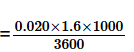= 0.0089 m/s

For the leopard, average speed = 70 mi/h =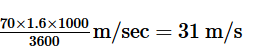Ques 8: The height of mercury column in a barometer in a Calcutta laboratory was recorded to be 75 cm. Calculate this pressure in SI and CGS units using the following data : Specific gravity of mercury = 13⋅6, Density of water=103 kg/m3, g= 9⋅8 m/s2 at Calcutta. Pressure =hρg in usual symbols.
Ans: Height, h = 75 cm = 0.75 m
Density of mercury = 13600 kg/m3
g = 9.8 m/s2
In SI units, pressure = hρg = 0.75 × 13600 × 9.8 = 10 × 104 N/m2 (approximately)
In CGS units, pressure = 10 × 104 N/m2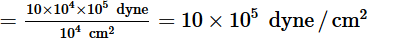Ques 9: Express the power of a 100 watt bulb in CGS unit.
Ans: In SI unit, watt = joule/s
In CGS unit, 1 joule = 107 erg

So, 100 watt = 100 joule/s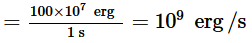Ques 10: The normal duration of I.Sc. Physics practical period in Indian colleges is 100 minutes. Express this period in microcenturies. 1 microcentury = 106 × 100 years. How many microcenturies did you sleep yesterday?
Ans: 1 microcentury = 10−6 × 100 years = 10−4 × 365 × 24 × 60 minutes

1 min =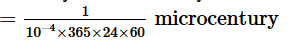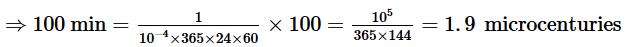Suppose, I slept x minutes yesterday.
x min = 0.019x microcenturies

Ques 11: The surface tension of water is 72 dyne/cm. Convert it in SI unit.
Ans: 1 dyne = 10−5 N
1 cm = 10−2 m
∴72 dyne/cm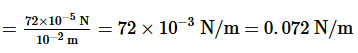Ques 12: The kinetic energy K of a rotating body depends on its moment of inertia I and its angular speed ω. Assuming the relation to be K=kIaωB where k is a dimensionless constant, find a and b. Moment of inertia of a sphere about its diameter is 2/5Mr2
Ans: Kinetic energy of a rotating body is K = kI aωb

Dimensions of the quantities are [K] = [ML2T−2], [I] = [ML2] and [ω] = [T−1].
Now, dimension of the right side are [I]a = [ML2]a and [ω]b = [T−1]b.
According to the principal of homogeneity of dimension, we have:
[ML2T−2] = [ML2]a [T−1]b
Equating the dimensions of both sides, we get:
2 = 2a
a = 1
And,
−2 = −b
b = 2

Ques 13: Theory of relativity reveals that mass can be converted into energy. The energy E so obtained is proportional to certain powers of mass m and the speed c of light. Guess a relation among the quantities using the method of dimensions.
Ans: According to the theory of relativity, E α macb
⇒ E = kmacb, where k = proportionality constant
Dimension of the left side, [E] = [ML2T−2]
Dimension of the right side, [macb]= [M]a [LT−1]b
Equating the dimensions of both sides, we get:
[ML2T−2] = [M]a [LT−1]b
a = 1, b = 2
E = kmc2

Ques 14: Let I = current through a conductor, R = its resistance and V = potential difference across its ends. According to Ohm's law, product of two of these quantities equals the third. Obtain Ohm's law from dimensional analysis. Dimensional formulae for R and V are ML2I−2T−3 and ML2T−3I−1 respectively.
Ans: Dimensional formula of resistance, [R] = [ML2A−2T−3]    ...(1)
Dimensional formula of potential difference, [V] = [ML2A−1T−3]    ...(2)
Dimensional formula of current,  = [A]
Dividing (2) by (1), we get: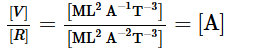⇒ V = IR

Ques 15: The frequency of vibration of a string depends on the length L between the nodes, the tension F in the string and its mass per unit length m. Guess the expression for its frequency from dimensional analysis.
Ans: Frequency, f ∝ LaFbmc
f = kLaFbmc   ...(1)
Dimension of [f] = [T−1]
Dimension of the right side components:
[L] = [L]
[F] = [MLT−2]
[m] = [ML−1]
Writing equation (1) in dimensional form, we get:
[T−1] = [L]a [MLT−2]b [ML−1]c
[M0L0T−1] = [Mb + c La + bc T−2b]
Equating the dimensions of both sides, we get:
b + c = 0               ....(i)
a + bc = 0      ....(ii)
−2b = −1              ....(iii)
Solving equations (i), (ii) and (iii), we get: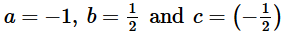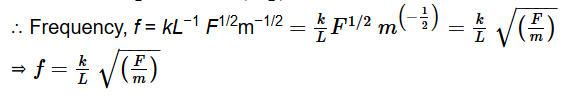Ques 16: Test if the following equations are dimensionally correct: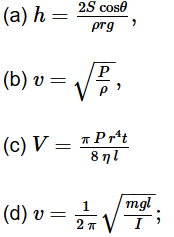where h = height, S = surface tension, ρ= density, P = pressure, V = volume, η= coefficient of viscosity, v = frequency and I = moment of interia.
Ans: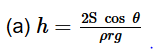Height, [h] = [L]
Surface Tension,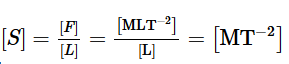Density,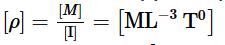Radius, [r] = [L], [g]= [LT−2

Now,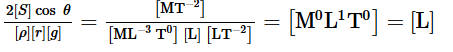Since the dimensions of both sides are the same, the equation is dimensionally correct.

(b)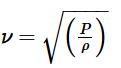Velcocity, [ν] = [LT−1]

Pressure, P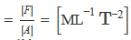Density ,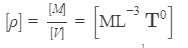Now,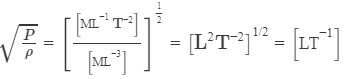Since the dimensions of both sides of the equation are the same, the equation is dimensionally correct.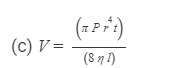Volume, [V] = [L3]
Pressure, P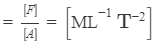[r]= [L] and [t] = [T]
Coefficient of viscosity,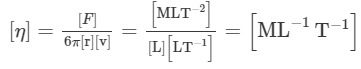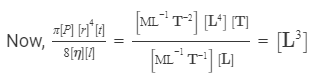Since the dimensions of both sides of the equation are the same, the equation is dimensionally correct.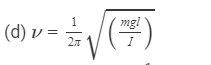Frequency, ν = [T−1]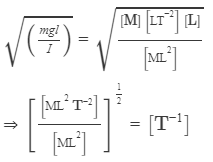Since the dimensions of both sides of the equation are the same, the equation is dimensionally correct.

Ques 17: Let x and a stand for distance. Is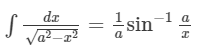dimensionally correct?
Ans: Dimension of the left side of the equation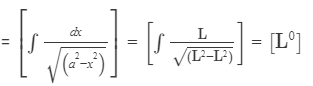Dimension of the right side of the equation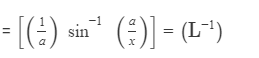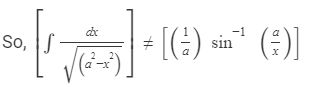Since the dimensions on both sides are not the same, the equation is dimensionally incorrect.

Offer running on EduRev: Apply code STAYHOME200 to get INR 200 off on our premium plan EduRev Infinity!

76 docs

,

,

,

,

,

,

,

,

,

,

,

,

,

,

,

,

,

,

,

,

,

;